Courses

# RD Sharma Solutions (Part - 2) - Ex-20.3, Mensuration - I, Class 7, Math Class 7 Notes | EduRev

## RD Sharma Solutions for Class 7 Mathematics

Created by: Abhishek Kapoor

## Class 7 : RD Sharma Solutions (Part - 2) - Ex-20.3, Mensuration - I, Class 7, Math Class 7 Notes | EduRev

The document RD Sharma Solutions (Part - 2) - Ex-20.3, Mensuration - I, Class 7, Math Class 7 Notes | EduRev is a part of the Class 7 Course RD Sharma Solutions for Class 7 Mathematics.
All you need of Class 7 at this link: Class 7

#### Question 11:

Find the area of a rhombus having each side equal to 15 cm and one of whose diagonals is 24 cm.

Let ABCD be the rhombus where diagonals intersect at O.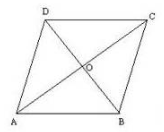Then AB = 15 cm and AC = 24 cm.
The diagonals of a rhombus bisect each other at right angles.
Therefore, Δ AOB is a right-angled triangle, right angled at O such that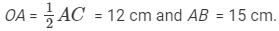By Pythagoras theorem, we have,
(AB)2(OA)2 + (OB)2
⇒ (15)2 = (12)2 + (OB)2
⇒ (OB)= (15)− (12)2
⇒ (OB)= 225 − 144 = 81
⇒ (OB)2 = (9)2
⇒ OB = 9 cm
∴ BD = 2 x OB = 2 x 9 cm = 18 cm
Hence,
Area of the rhombus ABCD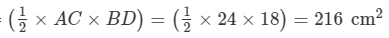#### Question 12:

Find the area of a rhombus, each side of which measures 20 cm and one of whose diagonals is 24 cm.

Let ABCD be the  rhombus whose diagonals intersect at O.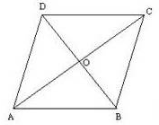Then AB = 20 cm and AC = 24 cm.
The diagonals of a rhombus bisect each other at right angles.
Therefore Δ AOB is a right-angled triangle, right angled at O such that
OA = 1/2AC = 12 cm and AB  = 20 cm
By Pythagoras theorem, we have,
(AB)2 = (OA)2 + (OB)2
⇒ (20)2 = (12)2 + (OB)2
⇒ (OB)= (20)− (12)2
⇒ (OB)= 400 − 144 = 256
⇒ (OB)2 = (16)2
OB = 16 cm
∴ BD = 2 x OB = 2 x 16 cm = 32 cm
Hence,
Area of the rhombus ABCD =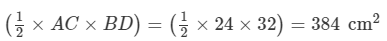#### Question 13:

The length of a side of a square field is 4 m. What will be the altitude of the rhombus, if the area of the rhombus is equal to the square field and one of its diagonals is 2 m?

We have,
Side of a square = 4 m and one diagonal of a square = 2 m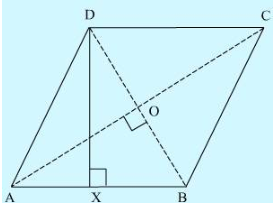Area of the rhombus = Area of the square of side 4 m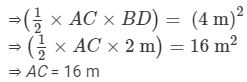We know that the diagonals of a rhombus are perpendicular bisectors of each other.

⇒ AO=1/2AC = 8 m  and BO =1/2BD=1 m
By Pythagoras theorem, we have:
AO2BO2AB2
AB2 = (8 m)2 + (1m)2 = 64 m2 + 1 m2 = 65 m2
⇒ Side of a rhombus = AB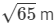Let DX be the altitude.
Area of the rhombus = AB × DX
16 m2x Dx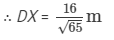Hence, the altitude of the rhombus will be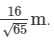#### Question 14:

Two sides of a parallelogram are 20 cm and 25 cm. If the altitude corresponding to the sides of length 25 cm is 10 cm, find the altitude corresponding to the other pair of sides.

We have,
ABCD is a parallelogram with longer side AB = 25 cm and  altitude AE = 10 cm.
As ABCD is a parallelogram .hence AB=CD    (opposite sides of parallelogram are equal)
The shorter side is AD =  20 cm and the corresponding altitude is CF.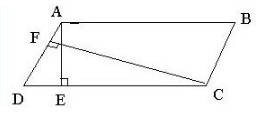Area of a parallelogram = Base × Height
We have two altitudes and two corresponding bases.
So,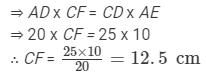Hence, the altitude corresponding to the other pair of the side AD is 12.5 cm.

#### Question 15:

The base and corresponding altitude of a parallelogram are 10 cm and 12 cm respectively. If the other altitude is 8 cm, find the length of the other pair of parallel sides.

We have,
ABCD is a parallelogram with side ABCD = 10 cm (Opposite sides of parallelogram are equal) and corresponding altitude AM = 12 cm.
The other side is AD and the corresponding altitude is CN = 8 cm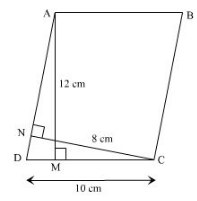Area of a parallelogram = Base × Height
We have two altitudes and two corresponding bases.
So,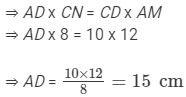Hence, the length of the other pair of the parallel side = 15 cm.

#### Question 16:

A floral design on the floor of a building consists of 280 tiles. Each tile is in the shape of a parallelogram of altitude 3 cm and base 5 cm. Find the cost of polishing the design at the rate of 50 paise per cm2.

We have,
Altitude of a tile = 3 cm
Base of a tile = 5 cm
Area of one tile = Altitude x Base = 5 cm x 3 cm = 15 cm2
Area of 280 tiles = 280 x 15 cm2 = 4200 cm2
Rate of polishing the tiles at 50 paise per cm2 = Rs. 0.5 per cm2
Thus,
Total cost of polishing the design = Rs. (4200 x 0.5) = Rs. 2100

97 docs

,

,

,

,

,

,

,

,

,

,

,

,

,

,

,

,

,

,

,

,

,

,

,

,

,

,

,

,

,

,

;